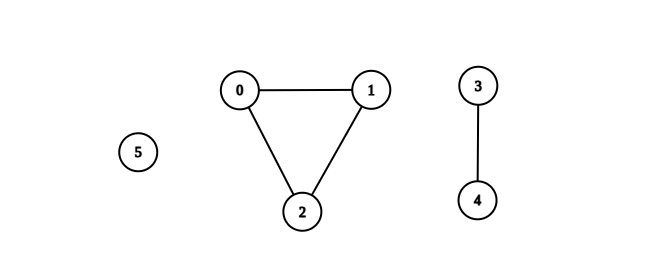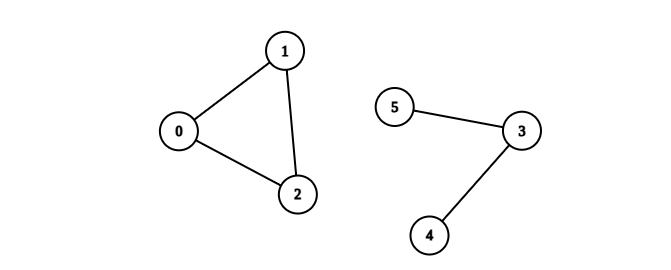2685. Count the Number of Complete Components
Medium
279
3

You are given an integer `n`. There is an undirected graph with `n` vertices, numbered from `0` to `n - 1`. You are given a 2D integer array `edges` where `edges[i] = [ai, bi]` denotes that there exists an undirected edge connecting vertices `ai` and `bi`.

Return the number of complete connected components of the graph.

A connected component is a subgraph of a graph in which there exists a path between any two vertices, and no vertex of the subgraph shares an edge with a vertex outside of the subgraph.

A connected component is said to be complete if there exists an edge between every pair of its vertices.

Example 1:```Input: n = 6, edges = [[0,1],[0,2],[1,2],[3,4]]
Output: 3
Explanation: From the picture above, one can see that all of the components of this graph are complete.
```

Example 2:```Input: n = 6, edges = [[0,1],[0,2],[1,2],[3,4],[3,5]]
Output: 1
Explanation: The component containing vertices 0, 1, and 2 is complete since there is an edge between every pair of two vertices. On the other hand, the component containing vertices 3, 4, and 5 is not complete since there is no edge between vertices 4 and 5. Thus, the number of complete components in this graph is 1.
```

Constraints:

• `1 <= n <= 50`
• `0 <= edges.length <= n * (n - 1) / 2`
• `edges[i].length == 2`
• `0 <= ai, bi <= n - 1`
• `ai != bi`
• There are no repeated edges.
Accepted
11.1K
Submissions
16.6K
Acceptance Rate
67.2%

Seen this question in a real interview before?
1/4
Yes
No

Discussion (0)

Related Topics